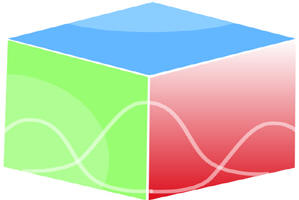``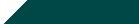Home| Journals | Statistical Calculator | About Us | Contact UsUntitled Document

Common Fixed Point Theorems for Sequence of Mappings in M - Fuzzy Metric Space

T. Veerapandi1, G. Uthaya Sankar2 and A. Subramanian3

1Associate Professor of Mathematics, P.M.T. College, Melaneelithanallur � 627953 INDIA

2Department of Mathematics, Mano College (An Institution of M.S. University, Tirunelveli), Sankarankovil � 627756 INDIA

3Department of Mathematics, The M.D.T. Hindu College, Tirunelveli � 627010 INDIA

1[email protected], 2[email protected], 3[email protected]

Research Article

Abstract: In this paper we prove some common fixed point theorems for sequence of mappings in complete   M � fuzzy metric space.

Keywords: Complete M � Fuzzy metric space, Common fixed point, Sequence of maps.

Mathematics Subject Classification: 47H10, 54H25.

1. Introduction and Preliminaries

Zadeh  introduced the concept of fuzzy sets in 1965. George and Veeramani  modified the concept of fuzzy metric space introduced by Kramosil and Michalek  and defined the Hausdorff topology of fuzzy metric spaces. Many authors [4, 7] have proved fixed point theorems in fuzzy metric space. Recently Sedghi and Shobe  introduced D*-metric space as a probable modification of the definition of D-metric introduced by Dhage , and prove some basic properties in D*-metric spaces. Using D*-metric concepts, Sedghi and Shobe define M � fuzzy metric space and proved a common fixed point theorem in it. In this paper we prove some common fixed point theorems for sequence of mappings in complete M � fuzzy metric space.

Definition 1.1: Let X be a nonempty set. A generalized metric (or D* - metric) on X is a function: D* : X3 [0, ), that satisfies the following conditions for each x, y, z, a X

(i)     D* (x, y, z) 0,

(ii)    D* (x, y, z) = 0   iff   x = y = z,

(iii) D* (x, y, z) = D* (p{x, y, z}), (symmetry) where p is a permutation function,

(iv)   D* (x, y, z) D* (x, y, a) + D* (a, z, z).

The pair (X, D*), is called a generalized metric (or D* - metric) space.

Example 1.2: Examples of  D* - metric are

(a)   D* (x, y, z) = max { d(x, y), d(y, z), d(z, x) },

(b)   D* (x, y, z) = d(x, y) + d(y, z) + d(z, x).

Here, d is the ordinary metric on X.

Definition 1.3: A fuzzy set M  in an arbitrary set X is a function with domain X and values in [0, 1].

Definition 1.4: A binary operation *: [0, 1] � [0, 1] [0, 1] is a continuous t-norm if it satisfies the following conditions

(i)    * is associative and commutative,

(ii)   * is continuous,

(iii)  a * 1 = a for all  a [0, 1],

(iv)  a*b c*d whenever a c and b d, for each a, b, c, d [0, 1].

Examples for continuous t-norm are a*b = ab and a*b = min {a, b}.

Definition 1.5: A 3-tuple (X, M, *) is called M � fuzzy metric space if X is an arbitrary non-empty set, * is a continuous t-norm, and M is a fuzzy set on X3 � (0, ), satisfying the following conditions for each x, y, z, a X and t, s > 0

(FM � 1 M (x, y, z, t) > 0

(FM � 2 M (x, y, z, t) = 1 iff  x = y = z

(FM � 3 M (x, y, z, t) = M (p {x, y, z}, t), where p is a permutation function

(FM � 4 M (x, y, a, t) * M (a, z, z, s) M (x, y, z, t+s)

(FM � 5 M (x, y, z, ) : (0, ) [0, 1] is continuous

(FM � 6limt M (x, y, z, t) = 1.

Example 1.6: Let X be a nonempty set and D* is the D* - metric on X. Denote a*b = a.b for all a, b [0, 1]. For each t (0, ), define

M (x, y, z, t) =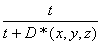for all x, y, z X, then (X, M, *) is a M � fuzzy metric space.

Example 1.7: Let (X, M, *) be a fuzzy metric space. If we define M : X3 � (0, ) [0, 1] by

M (x, y, z, t) = M (x, y, t)*M (y, z, t)*M (z, x, t)

for all x, y, z X, then (X, M, *) is a M � fuzzy metric space.

Lemma 1.8:() Let (X, M, *) be a M � fuzzy metric space. Then for every t > 0 and for every x, y X we have M(x, x, y, t) = M(x, y, y, t).

Lemma 1.9:() Let (X, M, *) be a M � fuzzy metric space. Then M (x, y, z, t) is non-decreasing with respect to t, for all x, y, z in X.

Definition 1.10: Let (X, M, *) be a M � fuzzy metric space. For t > 0, the open ball BM (x, r, t) with center x X and radius 0 < r < 1 is defined by

BM (x, r, t) = { y X: M (x, y, y, t) > 1 � r }.

A subset A of X is called open set if for each x A there exist t > 0 and 0 < r < 1 such that BM (x, r, t)   A.

Definition 1.11: Let (X, M, *) be a M � fuzzy metric space and {xn} be a sequence in X

(a)  {xn} is said to be converges to a point x X if limn M (x, x, xn, t) =1 for all t > 0

(b) {xn} is called Cauchy sequence if limn  M (xn+p, xn+p, xn, t) = 1 for all t > 0 and p > 0

(c) A M � fuzzy metric space in which every Cauchy sequence is convergent is said to be complete.

Remark 1.12: Since * is continuous, it follows from (FM-4) that the limit of the sequence is uniquely determined.Definition 1.13: Let (X, M, *) be a M � fuzzy metric space, then M is called of first type if for every x, y X we have

M (x, x, y, t) M (x, y, z, t)

for every z X. Also it is called of second type if for every x, y, z X we have

M (x, y, z, t) = M (x, y, t)*M (y, z, t)*M (z, x, t).

Example 1.14: Let X be a nonempty set and D* is the D* - metric on X. If we define

M (x, y, z, t) =,

where D*(x, y, z) = d(x, y) + d(y, z) + d(x, z), then M is first type.

Example 1.15: If (X, M, *) is a fuzzy metric and M (x, y, t) =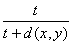, then

M (x, y, z, t) =*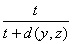*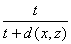is second type.

Definition 1.16: A point x X is said to be a fixed point of the map T: X X if Tx = x.

Definition 1.17: A point x X is said to be a common fixed point of sequence of maps Tn: X X if Tn(x) = x for all n.

2. Main Results

Theorem 2.1: Let (X, M, *) be a complete M � fuzzy metric space and Tn: X X be a sequence of maps such that for all t > 0 and 0 < k < 1 satisfying the condition

3M (Tix, Tjy, Tjy, t) ≥ {M (x, y, y, t/k) + M (x, x, Tix, t/k) + M (y, y, Tjy, t/k)}

for all ij and for all x, y X. Then {Tn} have a unique common fixed point.

Proof: Let x0 X be any arbitrary element.

Define a sequence {xn} in X as xn+1 = Tn+1xn for n = 0, 1, 2,�

Now we prove that {xn} is a Cauchy sequence in X.

For n 0, we have

3M (xn+1, xn+2, xn+2, t)

= 3M (Tn+1xn, Tn+2xn+1, Tn+2xn+1, t)

≥ {M (xn, xn+1, xn+1, t/k) + M (xn, xn, Tn+1xn, t/k)

+ M (xn+1, xn+1, Tn+2xn+1, t/k)}

= {M (xn, xn+1, xn+1, t/k) + M (xn, xn, xn+1, t/k)

+ M (xn+1, xn+1, xn+2, t/k)}

= 2M (xn, xn+1, xn+1, t/k) + M (xn+1, xn+2, xn+2, t/k)

2M (xn, xn+1, xn+1, t/k) + M (xn+1, xn+2, xn+2, t)

Therefore,

2M (xn+1, xn+2, xn+2, t) 2M (xn, xn+1, xn+1, t/k)

That is, M (xn+1, xn+2, xn+2, t) ≥ M (xn, xn+1, xn+1, t/k).

Continuing this way we get

M (xn+1, xn+2, xn+2, t) ≥ M (xn, xn+1, xn+1, t/k)

≥ M (x n-1, xn, xn, t/k2)

.

.

.

≥ M (x0, x1, x1, t/kn+1).

Now for any positive integer p and t > 0, we have

M (xn, xn+p, xn+p, t)

M (xn, xn+1, xn+1,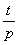)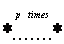M (xn+p-1, xn+p, xn+p,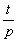)

M (x0, x1, x1,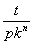)M (x0, x1, x1,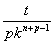)

Therefore, by (FM-6), we have

limn M (xn, xn+p, xn+p, t) 11 = 1

which implies that {xn} is a Cauchy sequence in M � fuzzy metric space X. Since X is M � fuzzy complete, sequence {xn} converges to a point x X.

Now we prove that x is a fixed point of {Tn} for all n.

Now we have

3M (Tmx, x, x, t) = limn   3M (Tmx, xn+2, xn+2, t)

= limn   3M (Tmx, Tn+2xn+1, Tn+2xn+1, t)

≥ limn   {M (x, xn+1, xn+1, t/k) + M (x, x, Tmx, t/k)

+ M (xn+1, xn+1, Tn+2xn+1, t/k)}

= limn   {M (x, xn+1, xn+1, t/k) + M (x, x, Tmx, t/k)

+ M (xn+1, xn+1, xn+2, t/k)}

= {M (x, x, x, t/k) + M (x, x, Tmx, t/k)

+ M (x, x, x, t/k)}

= {1 + M (x, x, Tmx, t/k) + 1}

= 2 + M (x, x, Tmx, t/k)

2 + M (x, x, Tmx, t)

Therefore, 2M (Tmx, x, x, t) 2

That is, M (Tmx, x, x, t) 1

Hence M (Tmx, x, x, t) = 1, for all t > 0.

Therefore, Tmx = x.

Hence Tnx = x for all n.

Therefore x is a common fixed point of {Tn}.

Uniqueness: Suppose xy such that Tny = y for all n.

Then

3M (x, y, y, t) = 3M (Tix, Tjy, Tjy, t)

≥ {M (x, y, y, t/k) + M (x, x, Tix, t/k)

+ M (y, y, Tjy, t/k)}

= {M (x, y, y, t/k) + M (x, x, x, t/k) + M (y, y, y, t/k)}

= M (x, y, y, t/k) + 2

M (x, y, y, t) + 2

Therefore, 2M (x, y, y, t) 2

That is, M (x, y, y, t) 1

Hence M (x, y, y, t) = 1, for all t > 0.

Therefore, x = y.

Hence {Tn} have a unique common fixed point.

This completes the proof.

Remark 2.2: From the above theorem we have,

M (Tix, Tjy, Tjy, t)

≥{M (x, y, y, t/k) + M (x, x, Tix, t/k)

+ M (y, y, Tjy, t/k)}

min {M (x, y, y, t/k), M (x, x, Tix, t/k),

M (y, y, Tjy, t/k)}

Therefore, M (Tix, Tjy, Tjy, t) min {M (x, y, y, t/k), M (x, x, Tix, t/k), M (y, y, Tjy, t/k)}.

Hence we get the following corollary.

Corollary 2.3: Let (X, M, *) be a complete M � fuzzy metric space and Tn: X X be a sequence of maps such that for all t > 0 and 0 < k < 1 satisfying the condition

M (Tix, Tjy, Tjy, t) min {M (x, y, y, t/k), M (x, x, Tix, t/k), M (y, y, Tjy, t/k)}

for all ij and for all x, y X. Then {Tn} have a unique common fixed point.

Remark 2.4: By taking Ti = Tj = T in the above corollary, we get the following corollary 2.5.

Corollary 2.5: Let (X, M, *) be a complete M � fuzzy metric space and let T: X X be a mapping such that for all t > 0 and 0 < k < 1 satisfying the condition

M (Tx, Ty, Ty, t) min {M (x, y, y, t/k), M (x, x, Tx, t/k), M (y, y, Ty, t/k)}

for all x, y X. Then T has a unique fixed point.

Theorem 2.6: Let (X, M, *) be a complete M � fuzzy metric space with continuous t-norm * is defined by a*b = min {a, b} and Tn: X X be a sequence of maps such that for all t > 0 and 0 < k < 1 satisfying the condition

M (Tix, Tjy, Tjy, t) ≥ {M (x, y, y, t/k)*M (x, x, Tix, t/k)*M (y, y, Tjy, t/k)}

for all ij and for all x, y X. Then {Tn} have a unique common fixed point.

Proof: Let x0 X be any arbitrary element.

Define a sequence {xn} in X as xn+1 = Tn+1xn for n = 0, 1, 2,�

Now we prove that {xn} is a Cauchy sequence in X.

For n 0, we have

M (xn+1, xn+2, xn+2, t) = M (Tn+1xn, Tn+2xn+1, Tn+2xn+1, t)

≥ {M (xn, xn+1, xn+1, t/k)*M (xn, xn, Tn+1xn, t/k)

*M (xn+1, xn+1, Tn+2xn+1, t/k)}

= {M (xn, xn+1, xn+1, t/k)*M (xn, xn, xn+1, t/k)

*M (xn+1, xn+1, xn+2, t/k)}

= {M (xn, xn+1, xn+1, t/k)*M (xn, xn+1, xn+1, t/k)

*M (xn+1, xn+2, xn+2, t/k)}

{M (xn, xn+1, xn+1, t/k)*M (xn+1, xn+2, xn+2, t/k)}

Therefore, M (xn+1, xn+2, xn+2, t) {M (xn, xn+1, xn+1, t/k)*M (xn+1, xn+2, xn+2, t/k)}, which implies that

M (xn+1, xn+2, xn+2, t) ≥ M (xn, xn+1, xn+1, t/k).

Continuing this way we get

M (xn+1, xn+2, xn+2, t) ≥ M (xn, xn+1, xn+1, t/k)

≥ M (x n-1, xn, xn, t/k2)

.

.

.

≥ M (x0, x1, x1, t/kn+1).

Now for any positive integer p and t > 0, we have

M (xn, xn+p, xn+p, t)

M (xn, xn+1, xn+1,)M (xn+p-1, xn+p, xn+p,)

M (x0, x1, x1,)M (x0, x1, x1,)

Therefore, by (FM-6), we have

limn M (xn, xn+p, xn+p, t) 11 = 1

which implies that {xn} is a Cauchy sequence in M � fuzzy metric space X. Since X is M � fuzzy complete, sequence {xn} converges to a point x X.

Now we prove that x is a fixed point of {Tn} for all n.

Now we have

M (Tmx, x, x, t) = limn   M (Tmx, xn+2, xn+2, t)

= limn   M (Tmx, Tn+2xn+1, Tn+2xn+1, t)

≥ limn   {M (x, xn+1, xn+1, t/k)*M (x, x, Tmx, t/k)

*M (xn+1, xn+1, Tn+2xn+1, t/k)}

= limn   {M (x, xn+1, xn+1, t/k)*M (x, x, Tmx, t/k)

*M (xn+1, xn+1, xn+2, t/k)}

= {M (x, x, x, t/k)*M (x, x, Tmx, t/k)*M (x, x, x, t/k)}

= {1*M (x, x, Tmx, t/k)*1}

= M (x, x, Tmx, t/k)

= M (Tmx, x, x, t/k)

.

.

.

≥ M (Tmx, x, x, t/kn)

1 as n

Hence M (Tmx, x, x, t) = 1, for all t > 0.

Therefore, Tmx = x.

Hence Tnx = x for all n.

Therefore x is a common fixed point of {Tn}.

Uniqueness: Suppose xy such that Tny = y for all n.

Then

M (x, y, y, t) = M (Tix, Tjy, Tjy, t)

≥ {M (x, y, y, t/k)*M (x, x, Tix, t/k)

*M (y, y, Tjy, t/k)}

= {M (x, y, y, t/k)*M (x, x, x, t/k)*M (y, y, y, t/k)}

= {M (x, y, y, t/k)*1*1}

= M (x, y, y, t/k)

.

.

.

M (x, y, y, t/kn)

1 as n

Hence M (x, y, y, t) = 1, for all t > 0.

Therefore, x = y.

Hence {Tn} have a unique common fixed point.

This completes the proof.

Remark 2.7: By taking Ti = Tj = T in the above theorem, we get the following corollary 2.8

Corollary 2.8: Let (X, M, *) be a complete M � fuzzy metric space with continuous t-norm * is defined by a*b = min {a, b} and let T: X X be a mapping such that for all t > 0 and 0 < k < 1 satisfying the condition

M (Tx, Ty, Ty, t) {M (x, y, y, t/k) * M (x, x, Tx, t/k)

* M (y, y, Ty, t/k)}

for all x, y X. Then T has a unique fixed point.

Theorem 2.9: Let (X, M, *) be a complete M � fuzzy metric space with continuous t-norm * is defined by a*b = min {a, b} and Tn: X X be a sequence of maps such that for all t > 0 and 0 < k < 1 satisfying the condition

M (Tix, Tjy, Tjy, t) ≥ min {M (x, y, y, t/k), M (x, x, Tix, t/k), M (y, y, Tjy, t/k), M (x, x, Tjy, 2t/k)}

for all ij and for all x, y X . Then {Tn} have a unique common fixed point.

Proof: Let x0 X be any arbitrary element.

Define a sequence {xn} in X as xn+1 = Tn+1xn for n = 0, 1, 2,�

Now we prove that {xn} is a Cauchy sequence in X.

For n 0, we have

M (xn+1, xn+2, xn+2, t) = M (Tn+1xn, Tn+2xn+1, Tn+2xn+1, t)

≥ min {M (xn, xn+1, xn+1, t/k), M (xn, xn, Tn+1xn, t/k),

M (xn+1, xn+1, Tn+2xn+1, t/k), M (xn, xn, Tn+2xn+1, 2t/k)}

= min {M (xn, xn+1, xn+1, t/k), M (xn, xn, xn+1, t/k),

M (xn+1, xn+1, xn+2, t/k), M (xn, xn, xn+2, 2t/k)}

= min {M (xn, xn+1, xn+1, t/k), M (xn, xn+1, xn+1, t/k),

M (xn+1, xn+2, xn+2, t/k), M (xn, xn, xn+2, 2t/k)}

= min {M (xn, xn+1, xn+1, t/k), M (xn+1, xn+2, xn+2, t/k),

M (xn, xn, xn+2, 2t/k)}

min {M (xn, xn+1, xn+1, t/k), M (xn+1, xn+2, xn+2, t/k),

M (xn, xn, xn+1, t/k)*M (xn+1, xn+2, xn+2, t/k)}

= {M (xn, xn+1, xn+1, t/k)*M (xn+1, xn+2, xn+2, t/k)}

Therefore, M (xn+1, xn+2, xn+2, t) {M (xn, xn+1, xn+1, t/k)*M (xn+1, xn+2, xn+2, t/k)}, which implies that

M (xn+1, xn+2, xn+2, t) ≥ M (xn, xn+1, xn+1, t/k).

Continuing this way we get

M (xn+1, xn+2, xn+2, t) ≥ M (xn, xn+1, xn+1, t/k)

≥ M (x n-1, xn, xn, t/k2)

.

.

.

≥ M (x0, x1, x1, t/kn+1).

Now for any positive integer p and t > 0, we have

M (xn, xn+p, xn+p, t)

M (xn, xn+1, xn+1,)M (xn+p-1, xn+p, xn+p,)

M (x0, x1, x1,)M (x0, x1, x1,)

Therefore, by (FM-6), we have

limn M (xn, xn+p, xn+p, t) 11 = 1

which implies that {xn} is a Cauchy sequence in M � fuzzy metric space X. Since X is M � fuzzy complete, sequence {xn} converges to a point x X.

Now we prove that x is a fixed point of {Tn} for all n.

Now we have

M (Tmx, x, x, t) = limn   M (Tmx, xn+2, xn+2, t)

= limn   M (Tmx, Tn+2xn+1, Tn+2xn+1, t)

≥ limn   min {M (x, xn+1, xn+1, t/k), M (x, x, Tmx, t/k), M (xn+1, xn+1, Tn+2xn+1, t/k), M (x, x, Tn+2xn+1, 2t/k)}

= limn   min {M (x, xn+1, xn+1, t/k), M (x, x, Tmx, t/k), M (xn+1, xn+1, xn+2, t/k), M (x, x, xn+2, 2t/k)}

= min {M (x, x, x, t/k), M (x, x, Tmx, t/k), M (x, x, x, t/k), M (x, x, x, 2t/k)}

= min {1, M (x, x, Tmx, t/k), 1, 1}

= M (x, x, Tmx, t/k)

= M (Tmx, x, x, t/k)

.

.

.

≥ M (Tmx, x, x, t/kn)

1 as n

Hence M (Tmx, x, x, t) = 1, for all t > 0.

Therefore, Tmx = x.

Hence Tnx = x for all n.

Therefore x is a common fixed point of {Tn}.

Uniqueness: Suppose xy such that Tny = y for all n.

Then

M (x, y, y, t) = M (Tix, Tjy, Tjy, t)

≥ min {M (x, y, y, t/k), M (x, x, Tix, t/k),

M (y, y, Tjy, t/k), M (x, x, Tjy, 2t/k)}

= min {M (x, y, y, t/k), M (x, x, x, t/k),

M (y, y, y, t/k), M (x, x, y, 2t/k)}

= min {M (x, y, y, t/k), 1, 1, M (x, y, y, 2t/k)}

= M (x, y, y, t/k)

.

.

.

M (x, y, y, t/kn)

1 as n

Hence M (x, y, y, t) = 1, for all t > 0.

Therefore, x = y.

Hence {Tn} have a unique common fixed point.

This completes the proof.

Remark 2.10: By taking Ti = Tj = T in the above theorem, we get the following corollary 2.11

Corollary 2.11: Let (X, M, *) be a complete M � fuzzy metric space with continuous t-norm * is defined by a*b = min {a, b} and let T: X X be a mapping such that for all t > 0 and 0 < k < 1 satisfying the condition

M (Tx, Ty, Ty, t) min {M (x, y, y, t/k), M (x, x, Tx, t/k), M (y, y, Ty, t/k), M (x, x, Ty, 2t/k)}

for all x, y X. Then T has a unique fixed point.

Theorem 2.12: Let (X, M, *) be a complete M � fuzzy metric space with continuous t-norm * is defined by a*b = min {a, b} and Tn: X X be a sequence of maps such that for all t > 0 and 0 < k < 1 satisfying the condition

M (Tix, Tjy, Tjy, t) ≥ {M (x, y, y, t/k)*M (x, x, Tix, t/k)*M (y, y, Tjy, t/k)*M (x, x, Tjy, 2t/k)}

for all ij and for all x, y X . Then {Tn} have a unique common fixed point.

Proof: Let x0 X be any arbitrary element.

Define a sequence {xn} in X as xn+1 = Tn+1xn for n = 0, 1, 2,�

Now we prove that {xn} is a Cauchy sequence in X.

For n 0, we have

M (xn+1, xn+2, xn+2, t) = M (Tn+1xn, Tn+2xn+1, Tn+2xn+1, t)

≥ {M (xn, xn+1, xn+1, t/k)*M (xn, xn, Tn+1xn, t/k)*

M (xn+1, xn+1, Tn+2xn+1, t/k)*M (xn, xn, Tn+2xn+1, 2t/k)}

= {M (xn, xn+1, xn+1, t/k)*M (xn, xn, xn+1, t/k)

*M (xn+1, xn+1, xn+2, t/k)*M (xn, xn, xn+2, 2t/k)}

= {M (xn, xn+1, xn+1, t/k)*M (xn, xn+1, xn+1, t/k)

*M (xn+1, xn+2, xn+2, t/k)*M (xn, xn, xn+2, 2t/k)}

{M (xn, xn+1, xn+1, t/k)*M (xn+1, xn+2, xn+2, t/k)

*M (xn, xn, xn+2, 2t/k)}

{M (xn, xn+1, xn+1, t/k)*M (xn+1, xn+2, xn+2, t/k)

*M (xn, xn, xn+1, t/k)*M (xn+1, xn+2, xn+2, t/k)}

{M (xn, xn+1, xn+1, t/k)*M (xn+1, xn+2, xn+2, t/k)}

Therefore, M (xn+1, xn+2, xn+2, t) {M (xn, xn+1, xn+1, t/k)*M (xn+1, xn+2, xn+2, t/k)}, which implies that

M (xn+1, xn+2, xn+2, t) ≥ M (xn, xn+1, xn+1, t/k).

Continuing this way we get

M (xn+1, xn+2, xn+2, t) ≥ M (xn, xn+1, xn+1, t/k)

≥ M (x n-1, xn, xn, t/k2)

.

.

.

≥ M (x0, x1, x1, t/kn+1).

Now for any positive integer p and t > 0, we have

M (xn, xn+p, xn+p, t)

M (xn, xn+1, xn+1,)M (xn+p-1, xn+p, xn+p,)

M (x0, x1, x1,)M (x0, x1, x1,)

Therefore, by (FM-6), we have

limn M (xn, xn+p, xn+p, t) 11 = 1

which implies that {xn} is a Cauchy sequence in M � fuzzy metric space X. Since X is M � fuzzy complete, sequence {xn} converges to a point x X.

Now we prove that x is a fixed point of {Tn} for all n.

Now we have

M (Tmx, x, x, t) = limn   M (Tmx, xn+2, xn+2, t)

= limn   M (Tmx, Tn+2xn+1, Tn+2xn+1, t)

≥ limn   {M (x, xn+1, xn+1, t/k)*M (x, x, Tmx, t/k)

*M (xn+1, xn+1, Tn+2xn+1, t/k)*M (x, x, Tn+2xn+1, 2t/k)}

= limn   {M (x, xn+1, xn+1, t/k)*M (x, x, Tmx, t/k)

*M (xn+1, xn+1, xn+2, t/k)*M (x, x, xn+2, 2t/k)}

= {M (x, x, x, t/k)*M (x, x, Tmx, t/k)*M (x, x, x, t/k)

*M (x, x, x, 2t/k)}

= {1*M (x, x, Tmx, t/k)*1*1}

= M (x, x, Tmx, t/k)

= M (Tmx, x, x, t/k)

.

.

.

≥ M (Tmx, x, x, t/kn)

1 as n

Hence M (Tmx, x, x, t) = 1, for all t > 0.

Therefore, Tmx = x.

Hence Tnx = x for all n.

Therefore x is a common fixed point of {Tn}.

Uniqueness: Suppose xy such that Tny = y for all n.

Then

M (x, y, y, t) = M (Tix, Tjy, Tjy, t)

≥ {M (x, y, y, t/k)*M (x, x, Tix, t/k)*M (y, y, Tjy, t/k)

*M (x, x, Tjy, 2t/k)}

= {M (x, y, y, t/k)*M (x, x, x, t/k)*M (y, y, y, t/k)

*M (x, x, y, 2t/k)}

= {M (x, y, y, t/k)*1*1*M (x, y, y, 2t/k)}

= {M (x, y, y, t/k)* M (x, y, y, 2t/k)}

{M (x, y, y, t/k)*M (x, y, y, t/k)}

M (x, y, y, t/k)

.

.

.

M (x, y, y, t/kn)

1 as n

Hence M (x, y, y, t) = 1, for all t > 0.

Therefore, x = y.

Hence {Tn} have a unique common fixed point.

This completes the proof.

Remark 2.13: By taking Ti = Tj = T in the above theorem, we get the following corollary 2.14

Corollary 2.14: Let (X, M, *) be a complete M � fuzzy metric space with continuous t-norm * is defined by a*b = min {a, b} and let T: X X be a mapping such that for all t > 0 and 0 < k < 1 satisfying the condition

M (Tx, Ty, Ty, t) {M (x, y, y, t/k) * M (x, x, Tx, t/k)

* M (y, y, Ty, t/k) * M (x, x, Ty, 2t/k)}

for all x, y X. Then T has a unique fixed point.

Theorem 2.15: Let (X, M, *) be a complete M � fuzzy metric space with continuous t-norm * is defined by a*b = min {a, b} and Tn: X X be a sequence of maps such that for all t > 0 and 0 < k < 1 satisfying the condition

M (Tix, Tjy, Tjy, t) ≥ min {M (x, y, y, t/k), M (x, Tix, Tjy, 2t/k)}

for all ij and for all x, y X . Then {Tn} have a unique common fixed point.

Proof: Let x0 X be any arbitrary element.

Define a sequence {xn} in X as xn+1 = Tn+1xn for n = 0, 1, 2,�

Now we prove that {xn} is a Cauchy sequence in X.

For n 0, we have

M (xn+1, xn+2, xn+2, t) = M (Tn+1xn, Tn+2xn+1, Tn+2xn+1, t)

min{M (xn, xn+1, xn+1, t/k), M (xn, Tn+1xn, Tn+2xn+1, 2t/k)}

= min {M (xn, xn+1, xn+1, t/k), M (xn, xn+1, xn+2, 2t/k)}

min {M (xn, xn+1, xn+1, t/k),

M (xn, xn+1, xn+1, t/k)*M (xn+1, xn+2, xn+2, t/k)}

= {M (xn, xn+1, xn+1, t/k)*M (xn+1, xn+2, xn+2, t/k)}

Therefore, M (xn+1, xn+2, xn+2, t) {M (xn, xn+1, xn+1, t/k)*M (xn+1, xn+2, xn+2, t/k)}, which implies that

M (xn+1, xn+2, xn+2, t) ≥ M (xn, xn+1, xn+1, t/k).

Continuing this way we get

M (xn+1, xn+2, xn+2, t) ≥ M (xn, xn+1, xn+1, t/k)

≥ M (x n-1, xn, xn, t/k2)

.

.

.

≥ M (x0, x1, x1, t/kn+1).

Now for any positive integer p and t > 0, we have

M (xn, xn+p, xn+p, t)

M (xn, xn+1, xn+1,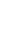)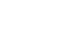M (xn+p-1, xn+p, xn+p,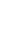)

M (x0, x1, x1,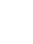)M (x0, x1, x1,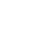)

Therefore, by (FM-6), we have

limn M (xn, xn+p, xn+p, t) 11 = 1

which implies that {xn} is a Cauchy sequence in M � fuzzy metric space X. Since X is M � fuzzy complete, sequence {xn} converges to a point x X.

Now we prove that x is a fixed point of {Tn} for all n.

Now we have

M (Tmx, x, x, t) = limn   M (Tmx, xn+2, xn+2, t)

= limn   M (Tmx, Tn+2xn+1, Tn+2xn+1, t)

limn min{M (x, xn+1, xn+1, t/k), M (x, Tmx, Tn+2xn+1, 2t/k)}

= limn   min {M (x, xn+1, xn+1, t/k), M (x, Tmx, xn+2, 2t/k)}

= min {M (x, x, x, t/k), M (x, Tmx, x, 2t/k)}

= min {1, M (Tmx, x, x, 2t/k)}

= M (Tmx, x, x, 2t/k)

M (Tmx, x, x, t/k)

.

.

.

M (Tmx, x, x, t/kn)

1 as n

Hence M (Tmx, x, x, t) = 1, for all t > 0.

Therefore, Tmx = x.

Hence Tnx = x for all n.

Therefore x is a common fixed point of {Tn}.

Uniqueness: Suppose xy such that Tny = y for all n.

Then

M (x, y, y, t) = M (Tix, Tjy, Tjy, t)

≥ min {M (x, y, y, t/k), M (x, Tix, Tjy, 2t/k)}

= min {M (x, y, y, t/k), M (x, x, y, 2t/k)}

= min { M (x, y, y, t/k), M (x, y, y, 2t/k)}

= M (x, y, y, t/k)

.

.

.

M (x, y, y, t/kn)

1 as n

Hence M (x, y, y, t) = 1, for all t > 0.

Therefore, x = y.

Hence {Tn} have a unique common fixed point.

This completes the proof.

Remark 2.16: By taking Ti = Tj = T in the above theorem, we get the following corollary 2.17

Corollary 2.17: Let (X, M, *) be a complete M � fuzzy metric space with continuous t-norm * is defined by a*b = min {a, b} and let T: X X be a mapping such that for all t > 0 and 0 < k < 1 satisfying the condition

M (Tx, Ty, Ty, t) min {M (x, y, y, t/k), M (x, Tx, Ty, 2t/k)}

for all x, y X. Then T has a unique fixed point.

Theorem 2.18: Let (X, M, *) be a complete first type M � fuzzy metric space and Tn: X X be a sequence of maps such that for all t > 0 and 0 < k < 1 satisfying the condition

3M (Tix, Tjy, Tkz, t) ≥ {M (x, y, z, t/k) + M (x, Tix, Tjy, t/k) + �[M (y, Tjy, Tkz, t/k)+M (z, Tkz, Tix, t/k)]}

for all ij k and for all x, y, z X . Then {Tn} have a unique common fixed point.

Proof: Let x0 X be any arbitrary element.

Define a sequence {xn} in X as xn+1 = Tn+1xn for n = 0, 1, 2,�

Now we prove that {xn} is a Cauchy sequence in X.

For n 0, we have

3M (xn+1, xn+2, xn+3, t)

= 3M (Tn+1xn, Tn+2xn+1, Tn+3xn+2, t)

{M (xn, xn+1, xn+2, t/k) + M (xn, Tn+1xn, Tn+2xn+1, t/k) + �[M(xn+1,Tn+2xn+1,Tn+3xn+2,t/k)+M(xn+2,Tn+3xn+2,Tn+1xn,t/k)]}

= {M (xn, xn+1, xn+2, t/k) + M (xn, xn+1, xn+2, t/k)

+ �[M (xn+1, xn+2, xn+3, t/k)+M (xn+2, xn+3, xn+1, t/k)]}

= {M (xn, xn+1, xn+2, t/k) + M (xn, xn+1, xn+2, t/k)

+ M (xn+1, xn+2, xn+3, t/k)}

= 2M (xn, xn+1, xn+2, t/k) + M (xn+1, xn+2, xn+3, t/k)

2M (xn, xn+1, xn+2, t/k) + M (xn+1, xn+2, xn+3, t)

Therefore,

2M (xn+1, xn+2, xn+3, t) 2M (xn, xn+1, xn+2, t/k)

That is, M (xn+1, xn+2, xn+3, t) M (xn, xn+1, xn+2, t/k).

Continuing this way we get

M (xn+1, xn+2, xn+3, t) M (xn, xn+1, xn+2, t/k)

M (xn-1, xn, xn+1, t/k2)

.

.

.

M (x0, x1, x2, t/kn+1)

1 as n

Since M is first type, we have

M (xn+1, xn+1, xn+2, t) M (xn+1, xn+2, xn+3, t)

Therefore, M (xn+1, xn+1, xn+2, t) 1 as n

Now for any positive integer p and t > 0, we have

M (xn, xn+p, xn+p, t)

M (xn, xn+1, xn+1,)M (xn+p-1, xn+p, xn+p,)

Taking limit as n ∞ we get

limn M (xn, xn+p, xn+p, t) 11 = 1

Therefore, limn M (xn, xn+p, xn+p, t) = 1

which implies that {xn} is a Cauchy sequence in M � fuzzy metric space X. Since X is M � fuzzy complete, sequence {xn} converges to a point x X.

Now we prove that x is a fixed point of {Tn} for all n.

Now we have

3M (Tmx, x, x, t) = limn   3M (Tmx, xn+2, xn+3, t)

= limn   3M (Tmx, Tn+2xn+1, Tn+3xn+2, t)

limn   {M (x, xn+1, xn+2, t/k) + M (x, Tmx, Tn+2xn+1, t/k) +�[M(xn+1,Tn+2xn+1,Tn+3xn+2, t/k)+M(xn+2,Tn+3xn+2,Tmx,t/k)]}

= limn {M (x, xn+1, xn+2, t/k) + M (x, Tmx, xn+2, t/k)

+ �[M (xn+1, xn+2, xn+3, t/k)+M (xn+2, xn+3, Tmx, t/k)]}

= {M (x, x, x, t/k) + M (x, Tmx, x, t/k)

+ � [M (x, x, x, t/k)+M (x, x, Tmx, t/k)]}

= {1 + M (Tmx, x, x, t/k) + �[1+M (Tmx, x, x, t/k)]}

= �[3M (Tmx, x, x, t/k) + 3]

6M (Tmx, x, x, t) 3M (Tmx, x, x, t/k) + 3

3M (Tmx, x, x, t) + 3

Therefore, 3M (Tmx, x, x, t) 3

That is, M (Tmx, x, x, t) 1

Hence M (Tmx, x, x, t) = 1, for all t > 0.

Therefore, Tmx = x.

Hence Tnx = x for all n.

Therefore x is a common fixed point of {Tn}.

Uniqueness: Suppose xy such that Tny = y for all n.

Then

3M (x, y, y, t) = 3M (Tix, Tjy, Tky, t)

{M (x, y, y, t/k) + M (x, Tix, Tjy, t/k)

+ �[M (y, Tjy, Tky, t/k)+M (y, Tky, Tix, t/k)]}

= {M (x, y, y, t/k) + M (x, x, y, t/k)

+ �[M (y, y, y, t/k)+M (y, y, x, t/k)]}

= {M (x, y, y, t/k) + M (x, y, y, t/k)

+ �[1+M (x, y, y, t/k)]}

= �[5M (x, y, y, t/k) + 1]

6M (x, y, y, t) 5M (x, y, y, t/k) + 1

5M (x, y, y, t) + 1

Therefore, M (x, y, y, t) 1

Hence M (x, y, y, t) = 1, for all t > 0.

Therefore, x = y.

Hence {Tn} have a unique common fixed point. This completes the proof.

Remark 2.19: From the above theorem we have,

M (Tix, Tjy, Tkz, t) ≥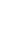{M (x, y, z, t/k) + M (x, Tix, Tjy, t/k) + �[M (y, Tjy, Tkz, t/k)+M (z, Tkz, Tix, t/k)]}

min {M (x, y, z, t/k), M (x, Tix, Tjy, t/k),

�[M (y, Tjy, Tkz, t/k)+M (z, Tkz, Tix, t/k)]}

Therefore,

M (Tix, Tjy, Tkz, t) ≥ min {M (x, y, z, t/k), M (x, Tix, Tjy, t/k), �[M (y, Tjy, Tkz, t/k)+M (z, Tkz, Tix, t/k)]}

Hence we get the following corollary.

Corollary 2.20: Let (X, M, *) be a complete first type M � fuzzy metric space and Tn: X X be a sequence of maps such that for all t > 0 and 0 < k < 1 satisfying the condition

M (Tix, Tjy, Tkz, t) ≥ min {M (x, y, z, t/k), M (x, Tix, Tjy, t/k), �[M (y, Tjy, Tkz, t/k)+M (z, Tkz, Tix, t/k)]}

for all ij k and for all x, y, z X . Then {Tn} have a unique common fixed point.

Remark 2.21: By taking Ti = Tj = Tk = T in the above corollary, we get the following corollary 2.22

Corollary 2.22: Let (X, M, *) be a complete first type M � fuzzy metric space and let T: X X be a mapping such that for all t > 0 and 0 < k < 1 satisfying the condition

M (Tx, Ty, Tz, t) ≥ min {M (x, y, z, t/k), M (x, Tx, Ty, t/k), �[M (y, Ty, Tz, t/k)+M (z, Tz, Tx, t/k)]}

for all x, y, z X. Then T has a unique fixed point.

Theorem 2.23: Let (X, M, *) be a complete first type M � fuzzy metric space and Tn: X X be a sequence of maps such that for all t > 0 and 0 < k < 1 satisfying the condition

3M (Tix, Tjy, Tkz, t) ≥ {M (x, y, z, t/k) + M (y, z, Tkz, t/k) + �[M (x, Tix, z, t/k)+M (x, y, Tjy, t/k)]}

for all ij k and for all x, y, z X . Then {Tn} have a unique common fixed point.

Proof: Let x0 X be any arbitrary element.

Define a sequence {xn} in X as xn+1 = Tn+1xn for n = 0, 1, 2,�

Now we prove that {xn} is a Cauchy sequence in X.

For n 0, we have

3M (xn+1, xn+2, xn+3, t)

= 3M (Tn+1xn, Tn+2xn+1, Tn+3xn+2, t)

{M (xn, xn+1, xn+2, t/k) + M (xn+1, xn+2, Tn+3xn+2, t/k)

+ �[M (xn, Tn+1xn, xn+2, t/k)+M (xn, xn+1, Tn+2xn+1, t/k)]}

= {M (xn, xn+1, xn+2, t/k) + M (xn+1, xn+2, xn+3, t/k)

+ �[M (xn, xn+1, xn+2, t/k)+M (xn, xn+1, xn+2, t/k)]}

= {M (xn, xn+1, xn+2, t/k) + M (xn+1, xn+2, xn+3, t/k)

+ M (xn, xn+1, xn+2, t/k)}

= 2M (xn, xn+1, xn+2, t/k) + M (xn+1, xn+2, xn+3, t/k)

2M (xn, xn+1, xn+2, t/k) + M (xn+1, xn+2, xn+3, t)

Therefore,

2M (xn+1, xn+2, xn+3, t) 2M (xn, xn+1, xn+2, t/k)

That is, M (xn+1, xn+2, xn+3, t) M (xn, xn+1, xn+2, t/k).

Continuing this way we get

M (xn+1, xn+2, xn+3, t) M (xn, xn+1, xn+2, t/k)

M (xn-1, xn, xn+1, t/k2)

.

.

.

M (x0, x1, x2, t/kn+1)

1 as n

Since M is first type, we have

M (xn+1, xn+1, xn+2, t) M (xn+1, xn+2, xn+3, t)

Therefore, M (xn+1, xn+1, xn+2, t) 1 as n

Now for any positive integer p and t > 0, we have

M (xn, xn+p, xn+p, t)

M (xn, xn+1, xn+1,)M (xn+p-1, xn+p, xn+p,)

Taking limit as n ∞ we get

limn M (xn, xn+p, xn+p, t) 11 = 1

Therefore, limn M (xn, xn+p, xn+p, t) = 1

which implies that {xn} is a Cauchy sequence in M � fuzzy metric space X. Since X is M � fuzzy complete, sequence {xn} converges to a point x X.

Now we prove that x is a fixed point of {Tn} for all n.

Now we have

3M (Tmx, x, x, t) = limn   3M (Tmx, xn+2, xn+3, t)

= limn   3M (Tmx, Tn+2xn+1, Tn+3xn+2, t)

limn {M (x, xn+1, xn+2, t/k) + M (xn+1, xn+2, Tn+3xn+2, t/k) + �[M (x, Tmx, xn+2, t/k)+M (x, xn+1, Tn+2xn+1, t/k)]}

= limn {M (x, xn+1, xn+2, t/k) + M (xn+1, xn+2, xn+3, t/k) + �[M (x, Tmx, xn+2, t/k)+M (x, xn+1, xn+2, t/k)]}

= {M (x, x, x, t/k) + M (x, x, x, t/k)

+ �[M (x, Tmx, x, t/k)+M (x, x, x, t/k)]}

= {1 + 1 + �[M (Tmx, x, x, t/k)+1]}

= �[M (Tmx, x, x, t/k) + 5]

6M (Tmx, x, x, t) M (Tmx, x, x, t/k) + 5

M (Tmx, x, x, t) + 5

Therefore, 5M (Tmx, x, x, t) 5

That is, M (Tmx, x, x, t) 1

Hence M (Tmx, x, x, t) = 1, for all t > 0.

Therefore, Tmx = x.

Hence Tnx = x for all n.

Therefore x is a common fixed point of {Tn}.

Uniqueness: Suppose xy such that Tny = y for all n.

Then

3M (x, y, y, t) = 3M (Tix, Tjy, Tky, t)

{M (x, y, y, t/k) + M (y, y, Tky, t/k)

+ �[M (x, Tix, y, t/k)+M (x, y, Tjy, t/k)]}

= {M (x, y, y, t/k) + M (y, y, y, t/k)

+ �[M (x, x, y, t/k)+M (x, y, y, t/k)]}

= {M (x, y, y, t/k) + 1

+ �[M (x, y, y, t/k)+M (x, y, y, t/k)]}

3M (x, y, y, t) 2M (x, y, y, t/k) + 1

2M (x, y, y, t) + 1

Therefore, M (x, y, y, t) 1

Hence M (x, y, y, t) = 1, for all t > 0.

Therefore, x = y. which is contradiction to xy.

Hence {Tn} have a unique common fixed point.

This completes the proof.

Remark 2.24: From the above theorem we have,

M (Tix, Tjy, Tkz, t) ≥{M (x, y, z, t/k) + M (y, z, Tkz, t/k) +�[M (x, Tix, z, t/k)+M (x, y, Tjy, t/k)]}

min {M (x, y, z, t/k), M (y, z, Tkz, t/k),

�[M (x, Tix, z, t/k)+M (x, y, Tjy, t/k)]}

Therefore,

M (Tix, Tjy, Tkz, t) min {M (x, y, z, t/k), M (y, z, Tkz, t/k), �[M (x, Tix, z, t/k)+M (x, y, Tjy, t/k)]}

Hence we get the following corollary.

Corollary 2.25: Let (X, M, *) be a complete first type M � fuzzy metric space and Tn: X X be a sequence of maps such that for all t > 0 and 0 < k < 1 satisfying the condition

M (Tix, Tjy, Tkz, t) min {M (x, y, z, t/k), M (y, z, Tkz, t/k), �[M (x, Tix, z, t/k)+M (x, y, Tjy, t/k)]}

for all ij k and for all x, y, z X . Then {Tn} have a unique common fixed point.

Remark 2.26: By taking Ti = Tj = Tk = T in the above corollary, we get the following corollary 2.27

Corollary 2.27: Let (X, M, *) be a complete first type M � fuzzy metric space and let T: X X be a mapping such that for all t > 0 and 0 < k < 1 satisfying the condition

M (Tx, Ty, Tz, t) min {M (x, y, z, t/k), M (y, z, Tz, t/k), �[M (x, Tx, z, t/k)+M (x, y, Ty, t/k)]}

for all x, y, z X . Then T has a unique fixed point.

Theorem 2.28: Let (X, M, *) be a complete first type M � fuzzy metric space and Tn: X X be a sequence of maps such that for all t > 0 and 0 < k < 1 satisfying the condition

5M (Tix, Tjy, Tkz, t) ≥ {M (x, y, z, t/k) + M (x, Tix, Tjy, t/k) + M (y, z, Tkz, t/k) + �[M (y, Tjy, Tkz, t/k)+M (z, Tkz, Tix, t/k)] + �[M (x, Tix, z, t/k)+M (x, y, Tjy, t/k)]}

for all ij k and for all x, y, z X . Then {Tn} have a unique common fixed point.

Proof: Let x0 X be any arbitrary element.

Define a sequence {xn} in X as xn+1 = Tn+1xn for n = 0, 1, 2,�

Now we prove that {xn} is a Cauchy sequence in X.

For n 0, we have

5M (xn+1, xn+2, xn+3, t)

= 5M (Tn+1xn, Tn+2xn+1, Tn+3xn+2, t)

{M (xn, xn+1, xn+2, t/k) + M (xn, Tn+1xn, Tn+2xn+1, t/k) + M (xn+1, xn+2, Tn+3xn+2, t/k) + �[M (xn+1,Tn+2xn+1,Tn+3xn+2,t/k)+M (xn+2,Tn+3xn+2,Tn+1xn,t/k) + �[M (xn, Tn+1xn, xn+2, t/k)+M (xn, xn+1, Tn+2xn+1, t/k)]}

={M(xn,xn+1,xn+2,t/k)+M(xn,xn+1,xn+2,t/k)

+M(xn+1,xn+2,xn+3, t/k)

+ �[M (xn+1, xn+2, xn+3, t/k)+M (xn+2, xn+3, xn+1, t/k)]

+ �[M (xn, xn+1, xn+2, t/k)+M (xn, xn+1, xn+2, t/k)]}

= {M (xn, xn+1, xn+2, t/k) + M (xn, xn+1, xn+2, t/k)

+ M (xn+1, xn+2, xn+3, t/k) + M (xn+1, xn+2, xn+3, t/k)

+ M (xn, xn+1, xn+2, t/k)}

= 3M (xn, xn+1, xn+2, t/k) + 2 M (xn+1, xn+2, xn+3, t/k)

3M (xn, xn+1, xn+2, t/k) + 2 M (xn+1, xn+2, xn+3, t)

Therefore,

3M (xn+1, xn+2, xn+3, t) 3M (xn, xn+1, xn+2, t/k)

That is, M (xn+1, xn+2, xn+3, t) M (xn, xn+1, xn+2, t/k).

Continuing this way we get

M (xn+1, xn+2, xn+3, t) M (xn, xn+1, xn+2, t/k)

M (xn-1, xn, xn+1, t/k2)

.

.

.

M (x0, x1, x2, t/kn+1)

1 as n

Since M is first type, we have

M (xn+1, xn+1, xn+2, t) M (xn+1, xn+2, xn+3, t)

Therefore, M (xn+1, xn+1, xn+2, t) 1 as n

Now for any positive integer p and t > 0, we have

M (xn, xn+p, xn+p, t)

M (xn, xn+1, xn+1,)M (xn+p-1, xn+p, xn+p,)

Taking limit as n ∞ we get

limn M (xn, xn+p, xn+p, t) 11 = 1

Therefore, limn M (xn, xn+p, xn+p, t) = 1

which implies that {xn} is a Cauchy sequence in M � fuzzy metric space X. Since X is M � fuzzy complete, sequence {xn} converges to a point x X.

Now we prove that x is a fixed point of {Tn} for all n.

Now we have

5M (Tmx, x, x, t) = limn   5M (Tmx, xn+2, xn+3, t)

= limn   5M (Tmx, Tn+2xn+1, Tn+3xn+2, t)

limn {M (x, xn+1, xn+2, t/k) + M (x, Tmx, Tn+2xn+1, t/k) + M (xn+1, xn+2, Tn+3xn+2, t/k) + �[M (xn+1,Tn+2xn+1,Tn+3xn+2,t/k) + M (xn+2,Tn+3xn+2,Tmx,t/k)] + �[M (x, Tmx, xn+2, t/k)+M (x, xn+1, Tn+2xn+1, t/k)]}

= limn {M (x, xn+1, xn+2, t/k)

+ M (x, Tmx, xn+2, t/k) + M (xn+1, xn+2, xn+3, t/k)

+ �[M (xn+1, xn+2, xn+3, t/k)+M (xn+2, xn+3, Tmx, t/k)]

+ �[M (x, Tmx, xn+2, t/k)+M (x, xn+1, xn+2, t/k)]}

= {M (x, x, x, t/k) + M (x, Tmx, x, t/k) + M (x, x, x, t/k)

+ �[M (x, x, x, t/k)+M (x, x, Tmx, t/k)]

+ �[M (x, Tmx, x, t/k)+M (x, x, x, t/k)]}

= {1 + M (Tmx, x, x, t/k) + 1 + �[1+M (Tmx, x, x, t/k)]

+ �[M (Tmx, x, x, t/k)+1]}

= 3 + 2M (Tmx, x, x, t/k)

3 + 2M (Tmx, x, x, t)

Therefore, 3M (Tmx, x, x, t) 3

That is, M (Tmx, x, x, t) 1

Hence M (Tmx, x, x, t) = 1, for all t > 0.

Therefore, Tmx = x.

Hence Tnx = x for all n.

Therefore x is a common fixed point of {Tn}.

Uniqueness: Suppose xy such that Tny = y for all n.

Then

5M (x, y, y, t) = 5M (Tix, Tjy, Tky, t)

{M (x, y, y, t/k) + M (x, Tix, Tjy, t/k)

+ M (y, y, Tky, t/k)

+ �[M (y, Tjy, Tky, t/k)+M (y, Tky, Tix, t/k)]

+ �[M (x, Tix, y, t/k)+M (x, y, Tjy, t/k)]}

= {M (x, y, y, t/k) + M (x, x, y, t/k) + M (y, y, y, t/k)

+ �[M (y, y, y, t/k)+M (y, y, x, t/k)]

+ �[M (x, x, y, t/k)+M (x, y, y, t/k)]}

= {M (x, y, y, t/k) + M (x, y, y, t/k) + 1

+ �[1+M (x, y, y, t/k)] + M (x, y, y, t/k)}

= �[7M (x, y, y, t/k) + 3]

10M (x, y, y, t) 7M (x, y, y, t/k) + 3

7M (x, y, y, t) + 3

Therefore, 3M (x, y, y, t) 3

That is, M (x, y, y, t) 1

Hence M (x, y, y, t) = 1, for all t > 0.

Therefore, x = y.

Hence {Tn} have a unique common fixed point.

This completes the proof.

Corollary 2.29: Let (X, M, *) be a complete first type M � fuzzy metric space and Tn: X X be a sequence of maps such that for all t > 0 and 0 < k < 1 satisfying the condition

M (Tix, Tjy, Tkz, t) ≥ min {M (x, y, z, t/k), M (x, Tix, Tjy, t/k), M (y, z, Tkz, t/k),�[M (y, Tjy, Tkz, t/k)+M (z, Tkz, Tix, t/k)], �[M (x, Tix, z, t/k)+M (x, y, Tjy, t/k)]}

for all ij k and for all x, y, z X . Then {Tn} have a unique common fixed point.

Remark 2.30: By taking Ti = Tj = Tk = T in the above corollary, we get the following corollary 2.31

Corollary 2.31: Let (X, M, *) be a complete first type M � fuzzy metric space and let T: X X be a mapping such that for all t > 0 and 0 < k < 1 satisfying the condition

M (Tx, Ty, Tz, t) ≥ min {M (x, y, z, t/k), M (x, Tx, Ty, t/k), M (y, z, Tz, t/k), �[M (y, Ty, Tz, t/k)+M (z, Tz, Tx, t/k)], �[M (x, Tx, z, t/k)+M (x, y, Ty, t/k)]}

for all x, y, z X . Then T has a unique fixed point.

3. References

        Dhage .B.C, Generalised metric spaces and mappings with fixed point, Bull. Calcutta Math. Soc., 84(4), (1992), 329-336.

        George .A and Veeramani .P, On some results in fuzzy metric space, Fuzzy Sets and Systems, 64 (1994), 395-399.

        Grabiec .M, Fixed points in fuzzy metric spaces, Fuzzy Sets and Systems, 27 (1988), 385-389.

        Gregori V and Sapena A, On fixed point theorem in fuzzy metric spaces, Fuzzy Sets and Systems, 125 (2002), 245-252.

        Klement .E.P, Mesiar .R and Pap .E, Triangular Norms, Kluwer Academic Publishers.

        Kramosil .I and Michalek .J, Fuzzy metric and statistical metric spaces, Kybernetica, 11 (1975), 326-334.

        Mihet D, A Banach contraction theorem in fuzzy metric spaces, Fuzzy Sets and Systems, 144 (2004), 431-439.

        Naidu .S.V.R, Rao .K.P.R and Srinivasa .R.N, On the topology of D-metric spaces and the generation of D-metric spaces from metric space, Internet. J. Math. Math. Sci., 51 (2004), 2719-2740.

        Naidu .S.V.R, Rao .K.P.R and Srinivasa .R.N, On the convergent sequences and fixed point theorems in D-metric spaces, Internet. J. Math. Math. Sci., 12 (2005), 1969-1988.

      Park .J.H, Park .J.S and Kwun .Y.C, Fixed point in M � fuzzy metric spaces, Optimization and Decision Making, 7 (2008), No.4, 305-315.

      Schweizer .B and Sklar .A, Statistical metric spaces, Pacific. J. Math., 10 (1960), 314-334.

      Sedghi .S and Shobe .N, A Common fixed point theorems in two M � fuzzy metric spaces, Commun. Korean Math. Soc. 22(2007), No.4, 513-526.

      Sedghi .S and Shobe .N, Fixed point theorem in M � fuzzy metric spaces with property (E), Advances in Fuzzy Mathematics, Vol.1, No.1 (2006), 55-65.

      Veerapandi .T, Jeyaraman .M and Paul Raj Josph .J, Some fixed point and coincident point theorem in generalized M � fuzzy metric space, Int. Journal of Math. Analysis, Vol.3, (2009), 627-635.

      Veeapandi .T, Uthaya Sankar .G and Subramanian .A, Some Common fixed point theorems in M � fuzzy metric space, Int. Journal of Mathematical Archive-3(3), (2012), 1005-1016.

      Zadeh .L. A, Fuzzy sets, Information and Control, 8 (1965), 338-353.